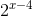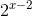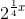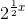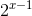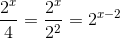# ISEE Upper Level Quantitative : How to find the length of the side of a square

## Example Questions

### Example Question #31 : Quadrilaterals

Which is the greater quantity?

(a) The sidelength of a square with area 400 square inches.

(b) The sidelength of a square with perimeter 80 inches.

(b) is greater

(a) and (b) are equal

It is impossible to tell which is greater from the information given

(a) is greater

(a) and (b) are equal

Explanation:

The sidelength of a square is the square root of its area and one-fourth of its perimeter, so:

(a) A square with area 400 square inches has sidelength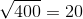inches.

(b) A square with perimeter 80 inches has sidelength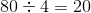inches.

The two quantities are equal.

### Example Question #2 : How To Find The Length Of The Side Of A Square

Which is the greater quantity?

(a) The sidelength of a square with area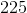square inches.

(b) The sidelength of a square with perimeterinches.

It is impossible to tell which is greater from the information given.

(a) and (b) are equal.

(b) is greater.

(a) is greater.

(a) is greater.

Explanation:

The sidelength of a square is the square root of its area and one-fourth of its perimeter, so:

(a) A square with areasquare inches has sidelength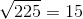inches.

(b) A square with perimeterinches has sidelength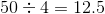inches.

(a) is the greater quantity.

### Example Question #3 : How To Find The Length Of The Side Of A Square

The perimeter of a square is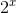. Give the length of each side in terms of.